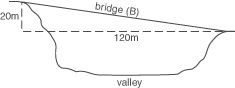Definition: Pythagoras’ theorem from The Penguin Dictionary of Science

The relationship a2 = b2 + c2 which holds between the lengths of the sides of any ➤right-angled triangle, where a is the length of the ➤hypotenuse and b and c are the lengths of the other two sides. Named after Pythagoras (6th century bc).

Summary Article: Pythagoras' theorem
From The Hutchinson Unabridged Encyclopedia with Atlas and Weather Guide

In geometry, a theorem stating that in a right-angled triangle, the square of the hypotenuse (the longest side) is equal to the sum of the squares of the other two sides. If the hypotenuse is h units long and the lengths of the other sides are a and b, then h2 = a2 + b2.

The theorem provides a way of calculating the length of any side of a right-angled triangle if the lengths of the other two sides are known. For example, to find the length of a bridge constructed over a valley 120 m wide, when the vertical drop of the bridge is 20 m:Using Pythagoras' theorem B2 = 1202 + 202, so B2 = 14,400 + 400 = 14,800, giving b = √14,800 = 121.66 (to two decimal places).

The length of the bridge is 121.66 m.

Pythagoras' theorem is also used to determine certain trigonometric identities such as sin2 θ + cos2 θ = 1.

essays

Mathematics

The Origins of Mathematics

Pythagoras' theorem: working out angles

At the Bottom of the Garden – Practical Use of Pythagoras' Theorem

It's That Man Again – Pythagoras and His Theorem

Dave's Short Trig Course

Pythagoras' Theorem

SOS Mathematics: Trigonometry

Trigonometry

images

Pythagoras' theorem© RM, 2018. All rights reserved.

### Related Articles

##### Full text Article sine
The Hutchinson Unabridged Encyclopedia with Atlas and Weather Guide

In trigonometry, a function of an angle in a right-angled triangle that is defined as the ratio of the length of the side opposite the angle to the l

##### Full text Article sine rule
The Hutchinson Unabridged Encyclopedia with Atlas and Weather Guide

The sine is a function of an angle in a right-angled triangle found by dividing the length of the side opposite the angle by the length of the

##### Full text Article The diagram shows a square-based pyramid. The sloping edges AT, BT, CT, and DT are all 15.6 m. The base has sides 4.7 m long. (a) Calculate angle ATD to the nearest degree. (b) Calculate the vertical distance from T to the base, giving your answer correct to two decimal places. (c) Calculate the angle between the base and the sloping sides, giving your answer to the nearest degree
The Hutchinson Unabridged Encyclopedia with Atlas and Weather Guide

Background The sides of a pyramid are isosceles triangles. An isosceles triangle has one line of symmetry that will divide the triangle into two rig

See more from Credo